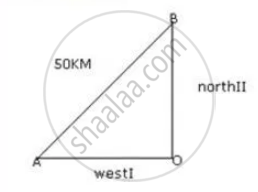Share

Two Trains Leave a Railway Station at the Same Time. the First Train Travels Due West and the Second Train Due North. the First Train Travels 5 Km/Hr Faster than the Second Train. If After 2 Hours - ICSE Class 10 - Mathematics

Question

Two trains leave a railway station at the same time. The first train travels due west and the second train due north. The first train travels 5 km/hr faster than the second train. If after 2 hours, they are 50 km apart, find the speed of each train.

Solution

Let the speed of the second train be x km/hr

Then the speed of the first train is (x + 5) km/hr

Let O be the position of the railways' station from which the two train leave

Distance travelled by the first train in 2 hours  = OA = Speed x Time = 2(x + 5) km

Distance travelled by the second train in 2 hours in OB = speed x Time = 2x kmBy Pythagoras Theorem we have

(AB)^2 = (OA)^2 + (OB)^2

=> (50)^2 = [2(x + 5)]^2 + (2x)^2

=> 2500 = 4(x + 5)^2 = 4x^2

=> 2500 = 4(x^2 + 25 + 10x) + 4x^2

=> 8x^2 + 40x - 2400 = 0

=> x^2 + 20x - 15x - 300 = 0

=> (x + 20)(x - 15) = 0

=> x = -20 or x = 15

=> x = 15 [∵ x  cannot be negative]

Hence the speed of the second train is 15 km/hr and the speed of the first train is 20 km/hr

Is there an error in this question or solution?

APPEARS IN

Selina Solution for Selina ICSE Concise Mathematics for Class 10 (2018-2019) (2017 to Current)
Chapter 6: Solving (simple) Problems (Based on Quadratic Equations)
Ex.6E | Q: 7

Video TutorialsVIEW ALL 

Solution Two Trains Leave a Railway Station at the Same Time. the First Train Travels Due West and the Second Train Due North. the First Train Travels 5 Km/Hr Faster than the Second Train. If After 2 Hours Concept: Quadratic Equations.
S